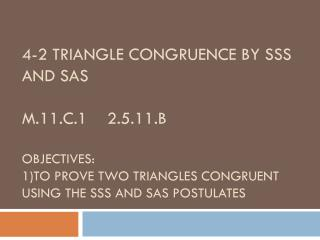DownloadDownload PresentationSide – Side – Side (SSS) Postulate

# Side – Side – Side (SSS) Postulate

Télécharger la présentation## Side – Side – Side (SSS) Postulate

- - - - - - - - - - - - - - - - - - - - - - - - - - - E N D - - - - - - - - - - - - - - - - - - - - - - - - - - -
##### Presentation Transcript

1. 4-2 Triangle congruence by SSS and sasM.11.C.1 2.5.11.BObjectives:1)to prove two triangles congruent using the SSS and SAS Postulates

2. Side – Side – Side (SSS) Postulate • If the three sides of one triangle are congruent to the three sides of another triangle, then the two triangles are congruent.

3. Side- Angle- Side (SAS) Postulate • If two sides and the included angle of one triangle are congruent to the two sides and the included angle of another triangle, then the two triangles are congruent.

4. Examples ___ ___ ___ • Given M is the midpoint of XY, AX ≅ AY • Can we prove △AMX ≅ △AMY? Explain.

5. Examples ___ ___ • AD ≅ BC • Prove △ADC ≅ △BCD two different ways.

6. Examples • ∠RSG ≅ ∠RSH • SG = SH • Can the given info prove △RSG ≅△RSH? ___ ___

7. Example • Can you prove the two triangles are congruent? • Triangle MNP and Triangle QRS with MN = QR, <N = <R and NP = RS.

8. CLASS WORK • Handed – In • Page 189 #1-5, 8-29